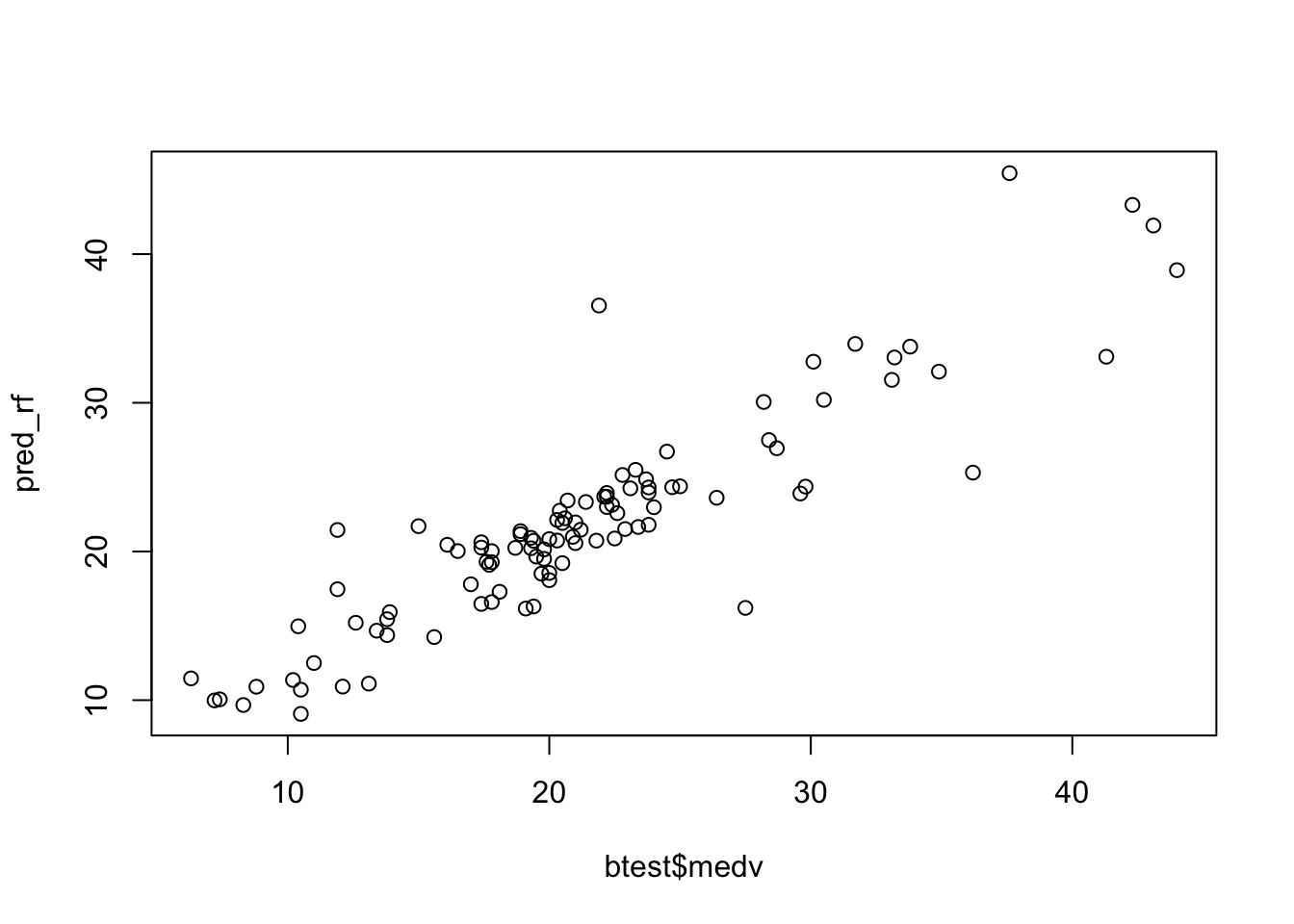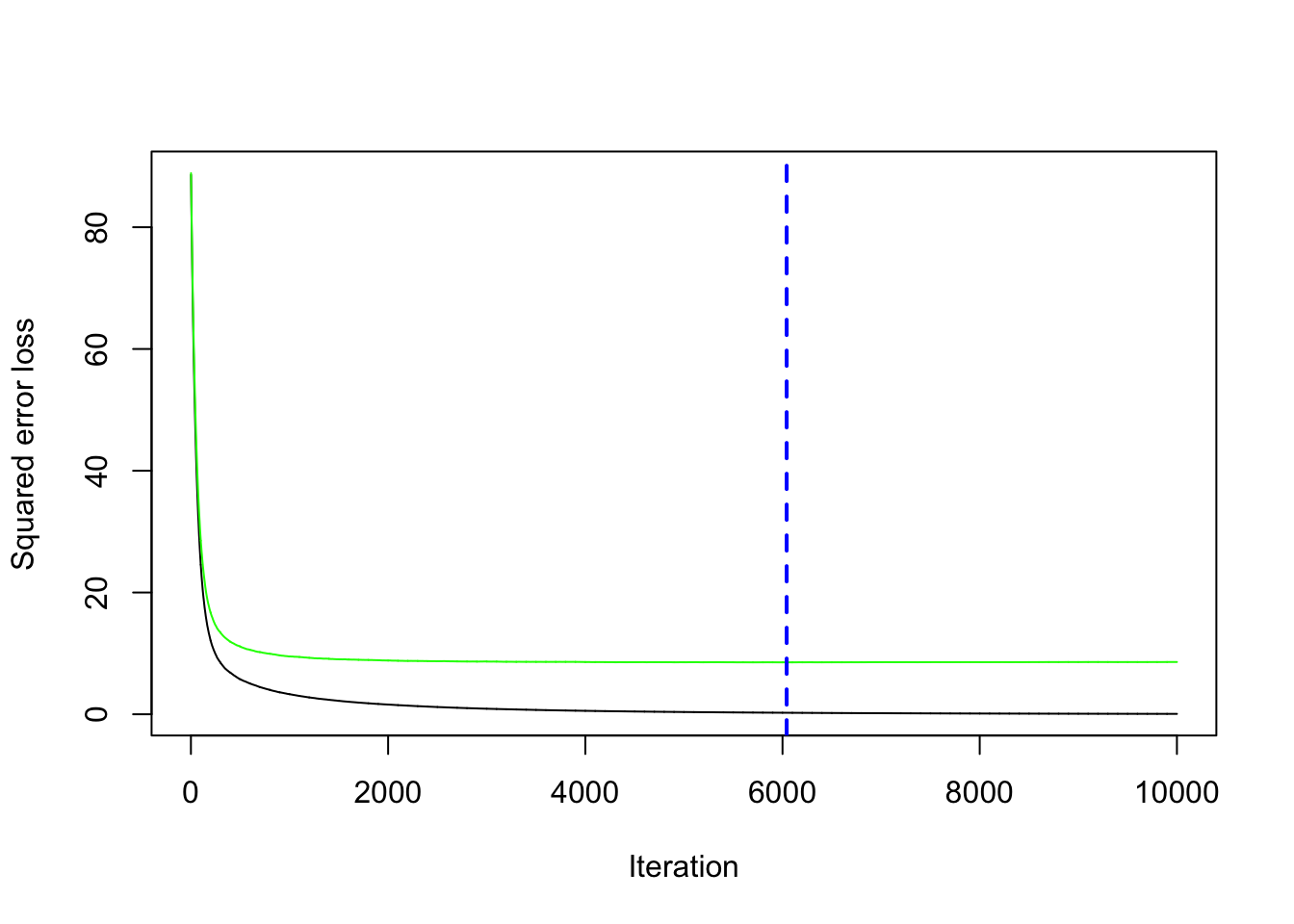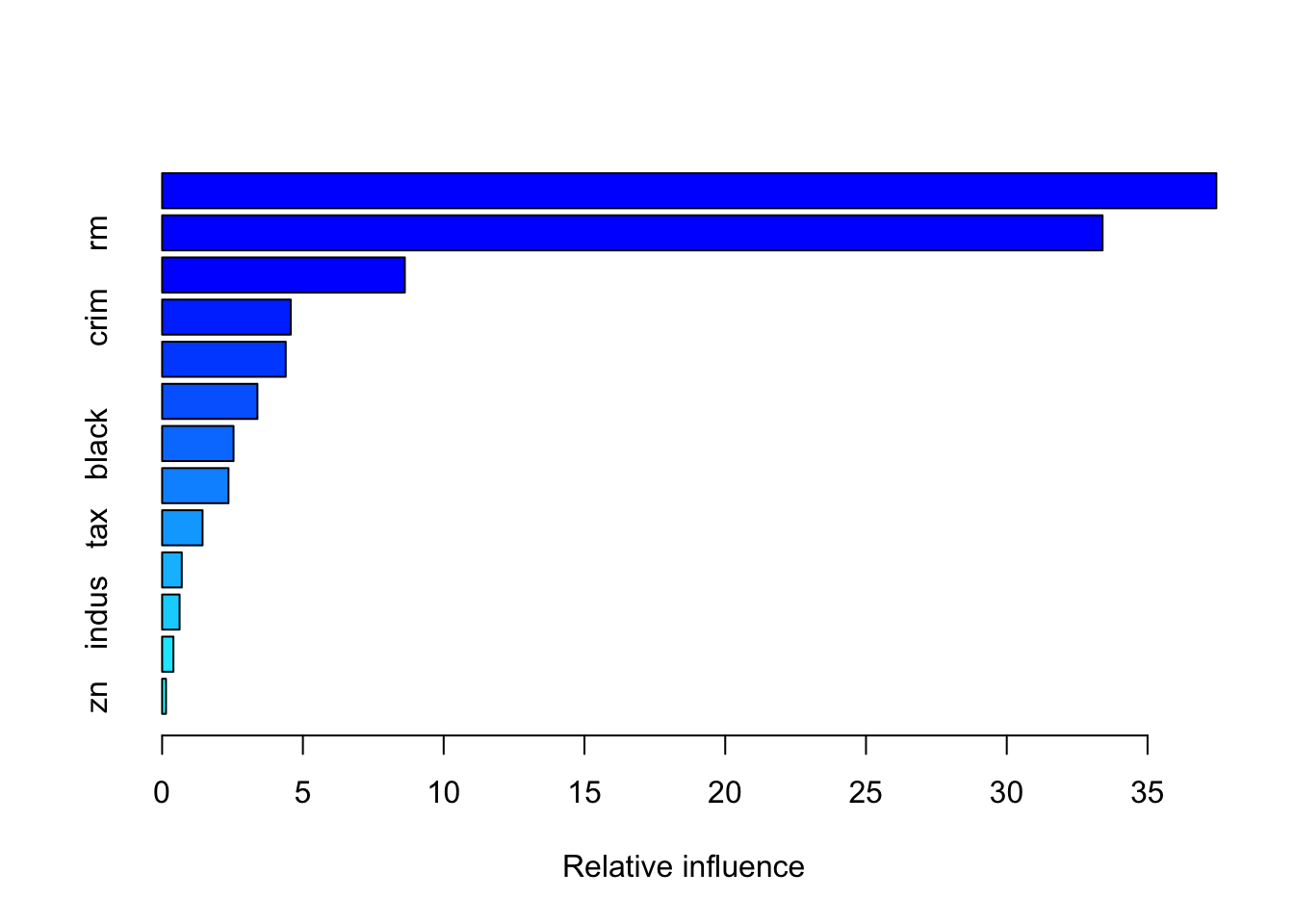# Chapter 6 R Lab 5 - 17/05/2021

In this lecture we will learn how to implement gradient boosting.

The following packages are required: MASS, randomForest, gbm and tidyverse.

library(MASS)
library(tidyverse)
library(randomForest) #for bagging and random forest
library(gbm) #for gradient boosting
## Loaded gbm 2.1.5

We first load the library MASS which contains the dataset Boston (Housing Values in Suburbs of Boston, see ?Boston for the description of the variables).

glimpse(Boston)
## Rows: 506
## Columns: 14
## $crim <dbl> 0.00632, 0.02731, 0.02729, 0.03237, 0.06905, 0.02985, 0.08829,… ##$ zn      <dbl> 18.0, 0.0, 0.0, 0.0, 0.0, 0.0, 12.5, 12.5, 12.5, 12.5, 12.5, 1…
## $indus <dbl> 2.31, 7.07, 7.07, 2.18, 2.18, 2.18, 7.87, 7.87, 7.87, 7.87, 7.… ##$ chas    <int> 0, 0, 0, 0, 0, 0, 0, 0, 0, 0, 0, 0, 0, 0, 0, 0, 0, 0, 0, 0, 0,…
## $nox <dbl> 0.538, 0.469, 0.469, 0.458, 0.458, 0.458, 0.524, 0.524, 0.524,… ##$ rm      <dbl> 6.575, 6.421, 7.185, 6.998, 7.147, 6.430, 6.012, 6.172, 5.631,…
## $age <dbl> 65.2, 78.9, 61.1, 45.8, 54.2, 58.7, 66.6, 96.1, 100.0, 85.9, 9… ##$ dis     <dbl> 4.0900, 4.9671, 4.9671, 6.0622, 6.0622, 6.0622, 5.5605, 5.9505…
## $rad <int> 1, 2, 2, 3, 3, 3, 5, 5, 5, 5, 5, 5, 5, 4, 4, 4, 4, 4, 4, 4, 4,… ##$ tax     <dbl> 296, 242, 242, 222, 222, 222, 311, 311, 311, 311, 311, 311, 31…
## $ptratio <dbl> 15.3, 17.8, 17.8, 18.7, 18.7, 18.7, 15.2, 15.2, 15.2, 15.2, 15… ##$ black   <dbl> 396.90, 396.90, 392.83, 394.63, 396.90, 394.12, 395.60, 396.90…
## $lstat <dbl> 4.98, 9.14, 4.03, 2.94, 5.33, 5.21, 12.43, 19.15, 29.93, 17.10… ##$ medv    <dbl> 24.0, 21.6, 34.7, 33.4, 36.2, 28.7, 22.9, 27.1, 16.5, 18.9, 15…

As usual we start by splitting the dataset into two subsets: one for training (called btrain and containing about 80% of the observations) and one for testing (called btest).

set.seed(1, sample.kind="Rejection")
ind = sample(1:nrow(Boston),0.8*nrow(Boston),replace=F)
btrain = Boston[ind,]
btest = Boston[-ind,]

## 6.1 Linear model, bagging and random forest

The response variable we are interested in is medv (median value of owner-occupied homes in $1000s). We implement first a linear regression model using as regressors all the other variables. Then we compute the predicted value for medv for the test set (btest) and finally compute the test mean square error (we save it in an object called MSE_lm). lm_boston = lm(medv ~ ., data=btrain) pred_lm = predict(lm_boston, newdata=btest) MSE_lm = mean((btest$medv - pred_lm)^2)
MSE_lm
##  17.33601

The second method we implement for predicting medv is bagging, described in Section 4.5 and 5.3. As previously done with the linear regression model, we compute the predicted value for medv for the test set (btest) and finally compute the test mean square error (we save it in an object called MSE_bag).

bag_boston = randomForest(formula = medv ~ .,
data = btrain,
mtry = (ncol(btrain)-1),
importance = T,
ntree = 1000)
pred_bag = predict(bag_boston, newdata=btest)
MSE_bag = mean((btest$medv - pred_bag)^2) MSE_bag ##  14.70622 Finally, we implement random forest as described in Section 5.4. We will compare this method with the previous two by using test mean square error (saved in an object called MSE_rf). rf_boston = randomForest(formula = medv ~ ., data = btrain, mtry = (ncol(btrain)-1)/3, importance = T, ntree = 1000) pred_rf = predict(rf_boston, newdata=btest) MSE_rf = mean((btest$medv - pred_rf)^2)
MSE_rf
##  11.3856

By comparing MSE_lm, MSE_bag and MSE_rf we can identify the best model implemented so far

MSE_lm
##  17.33601
MSE_bag
##  14.70622
MSE_rf
##  11.3856

It results that random forest performs the best because it has the lowest MSE.

Given the best method, we can then plot the observed and predicted value of medv and compute the correlation between observed and predicted value (the higher, the better):

plot(btest$medv, pred_rf)cor(btest$medv, pred_rf)
##  0.9031854

## 6.2 Gradient boosting: first run

We train a gradient boosting model (gbm) for predicting medv by using all the available regressors. Remember that with gbm there are 3 tuning parameters:

• $$B$$ the number of trees (i.e. iterations);
• the learning rate or shrinkage parameter $$\lambda$$;
• the maximum depth of each tree $$d$$ (interaction depth). Note that more than two splitting nodes are required to detect interactions between variables.

We start by setting $$B=5000$$ trees (n.trees = 5000), $$\lambda= 0.1$$ (shrinkage = 0.1) and $$d=1$$ (interaction.depth = 1, i.e. we consider only stumps). In order to study the effect of the tuning parameter $$B$$ we will use 5-folds cross-validation. Given that cross-validation is a random procedure we will set the seed before running the function gbm (see also ?gbm). In order to run the gradient boosting method we will have to specify also distribution = "gaussian":

set.seed(1, sample.kind="Rejection") #we use CV
gbm_boston = gbm(formula = medv ~ .,
data = btrain,
distribution = "gaussian",
n.trees = 5000, #B
shrinkage = 0.1, #lambda
interaction.depth = 1, #d
cv.folds = 5) 

We are now interested in exploring the content of the vector cv.error contained in the output object gbm_boston: it contains the values of the cv error (which is an estimate of the test error) for all the values of $$B$$ between 1 and 5000. For this reason the lenght of the vector is equal to $$B$$:

length(gbm_boston$cv.error) ##  5000 We want to discover which is the value of $$B$$ for which we obtaine the lowest value of the cv error: min(gbm_boston$cv.error) #lowest value of the cv error
##  12.10129
interaction.depth = hyper_grid$interaction.depth[i], #d cv.folds = 5) hyper_grid$minMSE[i] = min(gbm_boston$cv.error) hyper_grid$bestB[i] = which.min(gbm_boston$cv.error) } ##  "Iteration n. 1" ##  "Iteration n. 2" ##  "Iteration n. 3" ##  "Iteration n. 4" ##  "Iteration n. 5" ##  "Iteration n. 6" ##  "Iteration n. 7" ##  "Iteration n. 8" ##  "Iteration n. 9" We see that now hyper_grid contains two additional columns (minMSE and bestB). It is possible to order the data by using the values contained in the minMSE column in order to find the best value of $$\lambda$$ and $$d$$: hyper_grid ## shrinkage interaction.depth minMSE bestB ## 1 0.005 1 13.397062 5000 ## 2 0.010 1 12.554269 4960 ## 3 0.100 1 12.101286 832 ## 4 0.005 3 9.269789 4992 ## 5 0.010 3 8.788457 4891 ## 6 0.100 3 8.932166 543 ## 7 0.005 5 8.761203 4995 ## 8 0.010 5 8.537761 4923 ## 9 0.100 5 8.867994 216 hyper_grid %>% arrange(minMSE) ## shrinkage interaction.depth minMSE bestB ## 1 0.010 5 8.537761 4923 ## 2 0.005 5 8.761203 4995 ## 3 0.010 3 8.788457 4891 ## 4 0.100 5 8.867994 216 ## 5 0.100 3 8.932166 543 ## 6 0.005 3 9.269789 4992 ## 7 0.100 1 12.101286 832 ## 8 0.010 1 12.554269 4960 ## 9 0.005 1 13.397062 5000 Note that the optimal number of iterations and the learning rate $$\lambda$$ depend on each other (the lower the value of $$\lambda$$ the higher the number of iterations). ## 6.4 Gradient boosting: final model Given the best set of hyperparameters, we run the final gbm model with 10000 trees to see if we can get any additional improvement in the cv error. set.seed(1, sample.kind="Rejection") #we use CV gbm_final = gbm(formula = medv ~ ., data = btrain, distribution = "gaussian", n.trees = 10000, #B shrinkage = 0.01, #lambda interaction.depth = 5, #d cv.folds = 5) We save the best value of $$B$$ retrieved by using the gbm.perf function in a new object. This will be needed later for prediction: bestB = gbm.perf(gbm_final)We compute now the prediction for the test data using the standard predict function for which it will be necessary to specify the value of $$B$$ that we want to use (bestB in this case): pred_boo = predict(gbm_final, btest, n.trees = bestB) Given the prediction we can compute the MSE: MSE_boo = mean((btest$medv - pred_boo)^2)
MSE_boo
##  16.38891

We see that boosting performs poorly than random forest which is still the best method.

It is also possible to obtain information about the variable importance in the final model by applying the function summary:

summary(gbm_final)##             var    rel.inf
## lstat     lstat 37.4441271
## rm           rm 33.4032741
## dis         dis  8.6206651
## crim       crim  4.5679984
## nox         nox  4.3908304
## age         age  3.3853064
## black     black  2.5365640
## ptratio ptratio  2.3576062
## tax         tax  1.4367312
## zn           zn  0.1368894